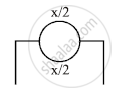# The Resistance of 11 is Connected in Right Gap. If Null Point is Obtained at a Distance of 45 Cm from the Left End, Find the Resistance of Metal Ring. - Physics

Two diametrically opposite points of a metal ring are connected to two terminals of the left gap of meter bridge. The resistance of 11 Ω is connected in right gap. If null point is obtained at a distance of 45 cm from the left end, find the resistance of metal ring.

#### Solution

R = 11Ω , lx = 45cm

lr=100-lx=55cmLet x be theresistance of metal ring

∴Equivalent resistance in left gap

1/R_p=1/(x

:. R_p=x/4

From balancing condition

:.R_p/R=l_x/(100-l_x)

(x

:.x/4=45/55xx11

∴ x=9x4

∴x=36Ω

Concept: Meter Bridge
Is there an error in this question or solution?
2013-2014 (March)

Share